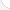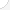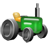Forest, conservation, and logging workersDescription: Forestry and conservation workers help develop and protect forests by planting new trees, fighting the pests and diseases that attack trees, and helping to control soil erosion. Timber cutters and loggers cut down thousands of acres of forests each year for the timber that is used for wood and paper products. Forestry, conservation, and logging workers work outdoors in all kinds of weather. Their work is demanding and dangerous.Source: Bureau of Labor Statistics, U.S. Department of Labor, Occupational Outlook Handbook, 2008-09 Edition at http://www.bls.gov/OCO/Complete Job Profile: http://www.bls.gov/oco/ocos178.htmSalary: \$23,001 to \$28,000 per yearComments:

There are 46 math topics Forest, conservation, and logging workers need to know.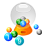Basic Math / Algebra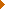FractionsDecimalsRatio and ProportionPercentCustomary MeasurementMetric MeasurementMeasurement ConversionBasic ProbabilityBasic StatisticsStatistical GraphingPowers and RootsNegative NumbersScientific NotationBasic Problem Solving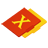First-Year AlgebraUsing FormulasLinear EquationsOperations with PolynomialsCoordinate Graphing 2DRadicalsQuadratic EquationsAlgebraic Representation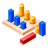GeometryBasic TerminologyAngle MeasurementCongruent TrianglesRight Triangle TrigonometryAreaVolumeMake/Use 3D Drawings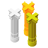Second-Year Algebra / TrigonometryFunctionsVariationLogarithmsSequences and SeriesMatricesCoordinate Graphing 3DAdvanced ProbabilityAdvanced StatisticsTrigonometric/Circular FunctionsGraphs of Trigonometric Functions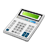Other TopicsBasic Calculator UseScientific Calculator UseComputer UseGroup Problem SolvingMental MathInductive/Deductive ReasoningMath CommunicationsMathematical Modeling